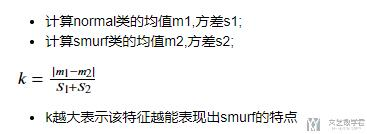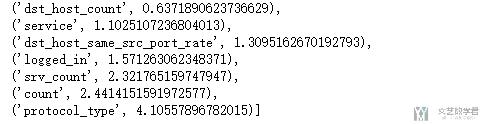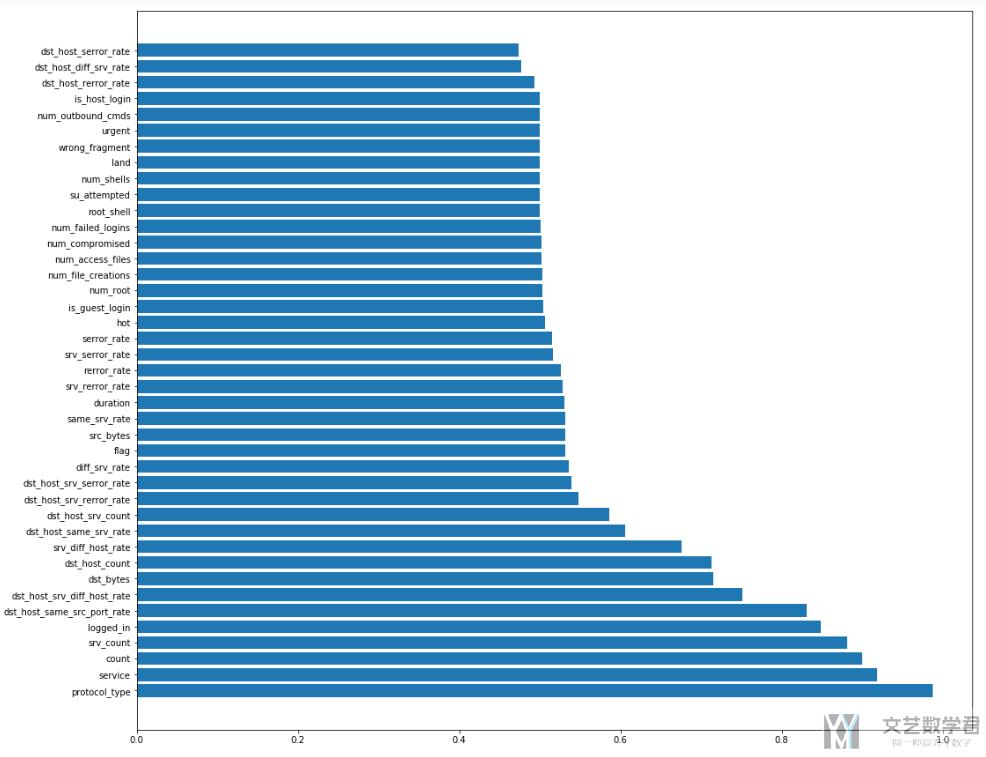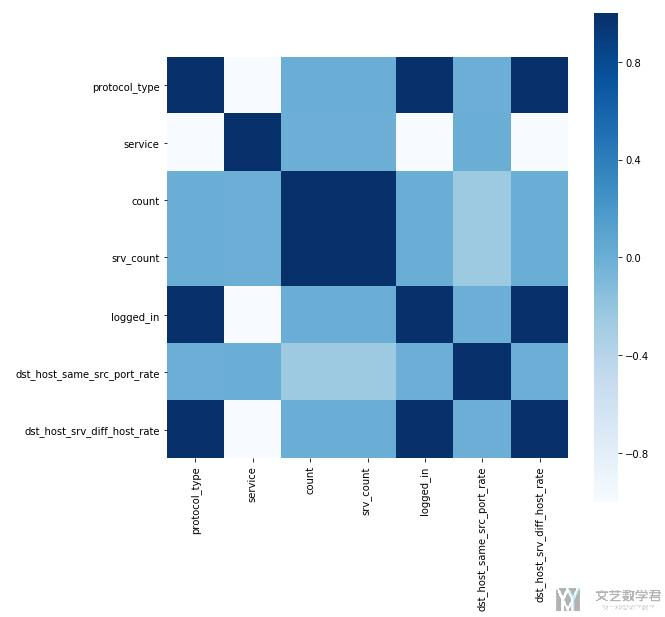# 数据关键特征的提取

• A+

## 方法介绍

### 类间距与类内方差• 可能是两类间距较大，即m1-m2的值比较大（分子比较大）
• 或是每类之间的方差比较小, 即每一类的特征都比较紧凑（分母比较小）
1. # DataProcessScale为原始数据集经过离散值处理和标准化之后的量
2. # 计算normal类的均值和方差
3. normalMean = DataProcessScale[DataProcessScale['labels']=='normal'].mean().values
4. normalVar = DataProcessScale[DataProcessScale['labels']=='normal'].var().values
5. # 计算smurf类的均值和方差
6. smurfMean = DataProcessScale[DataProcessScale['labels']=='smurf'].mean().values
7. smurfVar = DataProcessScale[DataProcessScale['labels']=='smurf'].var().values
8. # 计算k值, +0.0001防止分母为0
9. ks = abs(normalMean-smurfMean)/(normalVar+smurfVar+0.0001)
10. # 将k值与label对应, 保存为dict格式
11. k_value = {}
12. for name,k in zip(DataProcessScale.columns,ks):
13.     k_value[name]=k
14. # 将k值从大到小给出排序
15. sorted(k_value.items(), key=lambda d: d)

• smurf攻击主要是icmp协议
• 同时service主要是echo
• 同时是一种DoS攻击，所以count数会比较多### 单变量预测

1. from sklearn.linear_model import LogisticRegression
2. import seaborn as sns
3. import matplotlib.pyplot as plt
4. %matplotlib inline
5. # 找出labels
6. labels = pd.DataFrame((DataProcessScale['labels']=="normal").astype("int64"), columns=["labels"])
7. # 计算每个特征的准确率
8. accury = [] # 保存每个特征的准确率
9. columns_names = DataProcessScale.columns.values[:41]
10. for name in columns_names:
11.     data = DataProcessScale[name].values
12.     lr = LogisticRegression(solver='liblinear')
13.     lr.fit(data.reshape(-1,1), labels.values.squeeze())#进行拟合
14.     acc = lr.score(data.reshape(-1,1),labels.values.squeeze()) # 进行预测
15.     accury.append(acc) # 存储准确率
16. # 保存为字典类型
17. acc_name = {}
18. for name,acc in zip(columns_names,accury):
19.     acc_name[name]=acc
20. # 从大大小进行排序
21. acc_name_sort = sorted(acc_name.items(), key=lambda d: d, reverse=True)
22. # 进行绘图
23. fig = plt.figure(figsize=(17,15))
25. bar_data = [i for i in acc_name_sort]
26. bar_labels = [i for i in acc_name_sort]
27. plt.barh(range(len(bar_data)), bar_data, tick_label=bar_labels)
28. plt.show()• protocol_type : 协议类型(icmp)
• service : 目标主机的网络服务类型(ecr_i)
• count : 过去两秒内，与当前连接具有相同的目标主机的连接数
• srv_count : 过去两秒内，与当前连接具有相同服务的连接数
• logged_in : 成功登录则为1，否则为0(数据包内容)=>在smurf攻击中, 所有的logged_in都是0.
• dst_host_same_src_port_rate : 前100个连接中，与当前连接具有相同目标主机相同源端口的连接所占的百分比

### 相关系数计算

• protocol_type : 协议类型
• service : 目标主机的网络服务类型(Smurf攻击主要是icmp)
• count : 过去两秒内，与当前连接具有相同的目标主机的连接数
• srv_count : 过去两秒内，与当前连接具有相同服务的连接数
• logged_in : 成功登录则为1，否则为0(数据包内容)=>Smurf所有的logged都是0
• dst_host_same_src_port_rate : 前100个连接中，与当前连接具有相同目标主机相同源端口的连接所占的百分比=>Smurf所有的值都是0
• dst_host_srv_diff_host_rate : 前100个连接中，与当前连接具有相同目标主机相同服务的连接中，与当前连接具有不同源主机的连接所占的百分比=>Smurf所有的值都是0

1. corrData = DataProcessScale[DataProcessScale['labels']=='smurf'][['protocol_type','service','count','srv_count','logged_in','dst_host_same_src_port_rate','dst_host_srv_diff_host_rate']]
2. # 绘制图像
3. plt.subplots(figsize=(9, 9)) # 设置画面大小
4. sns.heatmap(corrData.corr(),square=True, cmap="Blues")## 结语• 微信公众号
• 关注微信公众号
•• QQ群
• 我们的QQ群号
•## 目前评论：1   其中：访客  0   博主  0

•赵倩

还有几种更常见的，比如算IV值，用随机森林之类的是吧？有一个问题，是为什么一定要用原始特征呢？原始特征的话，即使挑出来了强相关因子，也要考虑因子间共线性的吧？